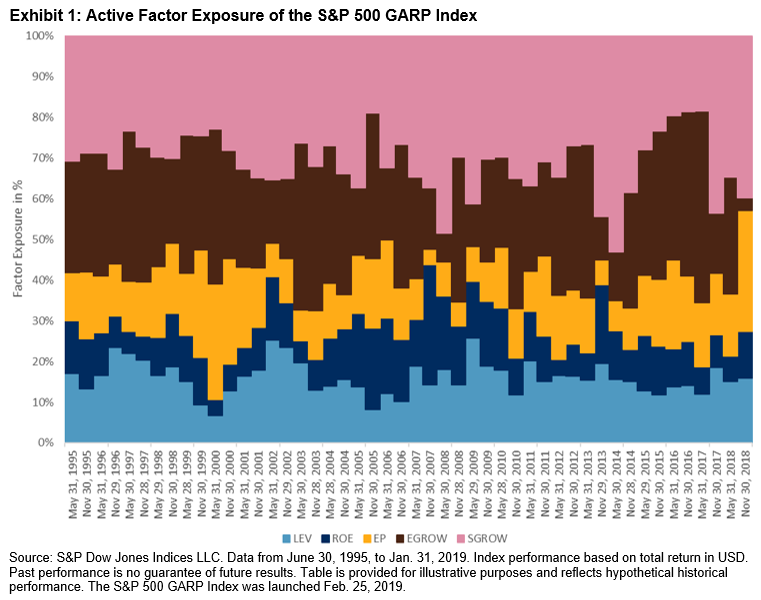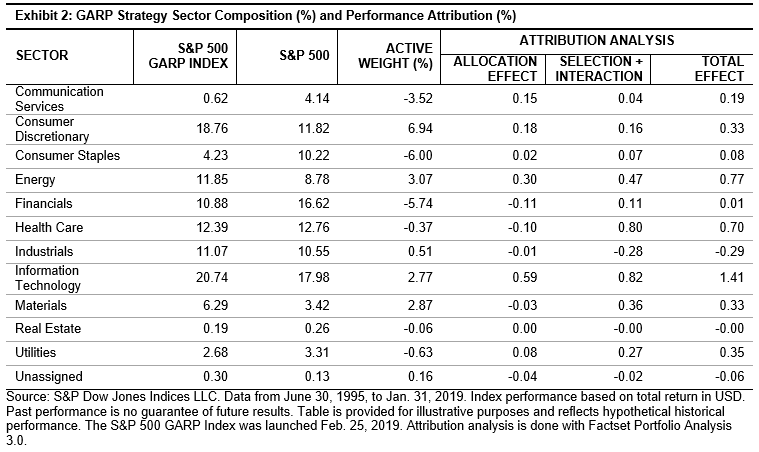# Using GARP Strategies for Indices Part IV – Factor Exposures, Sector Composition, and Performance Attribution

In this blog, the fourth in our introduction to Growth at a Reasonable Price (GARP) strategies, we cover factor exposures, sector composition, and performance attribution.

Targeted Factor Exposures

Exhibit 1 shows the active exposures (in percentages) of the S&P 500® GARP Index to the five factors used in GARP strategies: three-year sales per share (SPS) growth (SGROW), three-year earnings per share (EPS) growth (EGROW), earnings-to-price ratio (EP), return on equity (ROE), and financial leverage ratio (LEV). Active exposure is defined as the portfolio factor exposure minus the benchmark factor exposure. Factor percentage exposure is defined as the active exposure of one factor divided by the sum of all five factors. The monthly average of all periods is taken to represent the average active exposure. In Exhibit 1, we see that SPS growth and EPS growth had exposure levels that dominated the factor exposures, with 31% and 27%, respectively. EP, ROE, and LEV had exposures of 14%, 11%, and 16%, respectively. The factor exposure levels in the first layer of filters doubled that of the second layer of filters. The exposure results show that the multi-factor sequential filtering approach achieved its design goal.